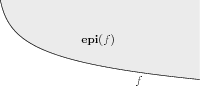# 12 Notation and definitions¶

$$\R$$ and $$\integral$$ denote the sets of real numbers and integers, respectively. $$\R^n$$ denotes the set of $$n$$-dimensional vectors of real numbers (and similarly for $$\integral^n$$ and $$\{0,1\}^n$$); in most cases we denote such vectors by lower case letters, e.g., $$a \in \R^n$$. A subscripted value $$a_i$$ then refers to the $$i$$-th entry in $$a$$, i.e.,

$a = (a_1, a_2, \dots, a_n).$

The symbol $$e$$ denotes the all-ones vector $$e=(1,\ldots,1)^T$$, whose length always follows from the context.

All vectors are interpreted as column-vectors. For $$a,b\in \R^n$$ we use the standard inner product,

$\langle a, b \rangle := a_1 b_1 + a_2 b_2 + \dots + a_n b_n,$

which we also write as $$a^T b := \langle a, b\rangle$$. We let $$\R^{m\times n}$$ denote the set of $$m\times n$$ matrices, and we use upper case letters to represent them, e.g., $$B\in \R^{m\times n}$$ is organized as

$\begin{split}B = \left[\begin{array}{cccc} b_{11} & b_{12} & \dots & b_{1n}\\ b_{21} & b_{22} & \dots & b_{2n}\\ \vdots & \vdots & \ddots & \vdots\\ b_{m1} & b_{m2} & \dots & b_{mn} \end{array} \right]\end{split}$

For matrices $$A,B\in \R^{m\times n}$$ we use the inner product

$\langle A, B \rangle := \sum_{i=1}^m \sum_{j=1}^n a_{ij} b_{ij}.$

For a vector $$x\in \R^n$$ we have

$\begin{split}\mathbf{Diag}(x) := \left[\begin{array}{cccc} x_1 & 0 & \dots & 0\\ 0 & x_2 & \dots & 0\\ \vdots & \vdots & \ddots & \vdots \\ 0 & 0 & \dots & x_n \end{array} \right],\end{split}$

i.e., a square matrix with $$x$$ on the diagonal and zero elsewhere. Similarly, for a square matrix $$X\in\R^{n\times n}$$ we have

$\mathbf{diag}(X) := (x_{11}, x_{22}, \dots, x_{nn}).$

A set $$S\subseteq \R^n$$ is convex if and only if for any $$x, y\in S$$ and $$\theta\in[0,1]$$ we have

$\theta x + (1-\theta) y \in S$

A function $$f:\R^n \mapsto \R$$ is convex if and only if its domain $$\mathbf{dom}(f)$$ is convex and for all $$\theta \in [0,1]$$ we have

$f(\theta x + (1-\theta)y) \leq \theta f(x) + (1-\theta) f(y).$

A function $$f:\R^n \mapsto \R$$ is concave if and only if $$-f$$ is convex. The epigraph of a function $$f:\R^n \mapsto \R$$ is the set

$\mathbf{epi}(f) := \{ (x,t) \mid x \in \mathbf{dom}(f), \: f(x) \leq t \},$

shown in Fig. 12.1.Fig. 12.1 The shaded region is the epigraph of the function $$f(x)=-\log(x)$$.¶

Thus, minimizing over the epigraph

$\begin{split}\begin{array}{ll} \mbox{minimize}& t\\ \mbox{subject to}& f(x) \leq t \end{array}\end{split}$

is equivalent to minimizing $$f(x)$$. Furthermore, $$f$$ is convex if and only if $$\mathbf{epi}(f)$$ is a convex set. Similarly, the hypograph of a function $$f:\R^n \mapsto \R$$ is the set

$\mathbf{hypo}(f) := \{ (x,t) \mid x\in \mathbf{dom}(f),\: f(x) \geq t \}.$

Maximizing $$f$$ is equivalent to maximizing over the hypograph

$\begin{split}\begin{array}{ll} \mbox{maximize} & t\\ \mbox{subject to} & f(x) \geq t, \end{array}\end{split}$

and $$f$$ is concave if and only if $$\mathbf{hypo}(f)$$ is a convex set.